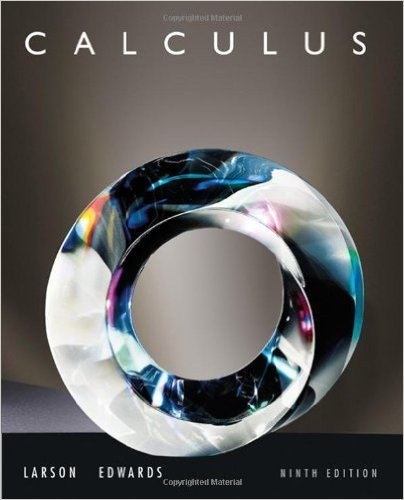×
Log in to StudySoup
Get Full Access to Calculus - Textbook Survival Guide
Join StudySoup for FREE
Get Full Access to Calculus - Textbook Survival Guide

Already have an account? Login here
×
Reset your password

# Solutions for Chapter 2: Differentiation## Full solutions for Calculus | 9th Edition

ISBN: 9780547167022Solutions for Chapter 2: Differentiation

Solutions for Chapter 2
4 5 0 276 Reviews
10
3
##### ISBN: 9780547167022

This textbook survival guide was created for the textbook: Calculus , edition: 9. This expansive textbook survival guide covers the following chapters and their solutions. Since 115 problems in chapter 2: Differentiation have been answered, more than 109654 students have viewed full step-by-step solutions from this chapter. Chapter 2: Differentiation includes 115 full step-by-step solutions. Calculus was written by and is associated to the ISBN: 9780547167022.

Key Calculus Terms and definitions covered in this textbook
• Angular speed

Speed of rotation, typically measured in radians or revolutions per unit time

• Aphelion

The farthest point from the Sun in a planet’s orbit

• Characteristic polynomial of a square matrix A

det(xIn - A), where A is an n x n matrix

• Cycloid

The graph of the parametric equations

• Demand curve

p = g(x), where x represents demand and p represents price

• Divergence

A sequence or series diverges if it does not converge

• Frequency (in statistics)

The number of individuals or observations with a certain characteristic.

• Horizontal component

See Component form of a vector.

• Imaginary part of a complex number

See Complex number.

• Limaçon

A graph of a polar equation r = a b sin u or r = a b cos u with a > 0 b > 0

• Odd function

A function whose graph is symmetric about the origin (ƒ(-x) = -ƒ(x) for all x in the domain of f).

• Polar axis

See Polar coordinate system.

• Rectangular coordinate system

See Cartesian coordinate system.

• Scalar

A real number.

• Slope

Ratio change in y/change in x

• Symmetric matrix

A matrix A = [aij] with the property aij = aji for all i and j

• Terminal side of an angle

See Angle.

• Triangular number

A number that is a sum of the arithmetic series 1 + 2 + 3 + ... + n for some natural number n.

• Upper bound for real zeros

A number d is an upper bound for the set of real zeros of ƒ if ƒ(x) ? 0 whenever x > d.

• Window dimensions

The restrictions on x and y that specify a viewing window. See Viewing window.